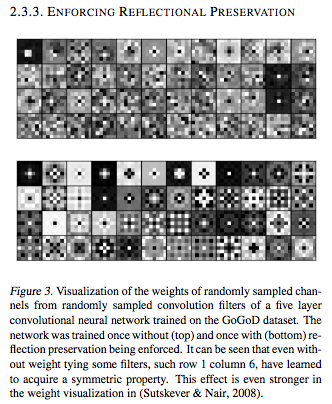# Deep learning for&#8230; Go

This is the last post about deep learning for chess/go/whatever. But this really cool paper by Christopher Clark and Amos Storkey was forwarded to me by Michael Eickenberg. It's about using convolutional neural networks to play Go. The authors of the paper do a much better job than I would ever have done of modeling move prediction in Go and show that their model beat certain Go engines.

The fascinating thing about this paper is that playing against other Go engines, they just plug in their move prediction function, with no deep search beyond one level. That means the total time it spends is a fraction of the opponents. Still, the fact that it plays so well speaks for its strength.

So what happened if we could plug this into a deep search framework? The authors suggest doing exactly that in the conclusion. State of the art of Go engines actually use Monte Carlo tree search rather than minimax but other than that, it's the same principle.

I talked a bit with the authors and the main thing that you have to change is to switch from move prediction to an evaluation function. For my chess experiments, I found a (hacky) way to train a function that does both at the same time. There's essentially two terms in my objective function: one is comparing the actual move with a random move, using a sigmoid:

$$frac{P(q)}{P(q) + P(r)} = frac{exp(f(q))}{exp(f(q)) + exp(f(r))}$$ .

If you extend that to all possible random moves you actually get a full probability distribution (a softmax) over all possible next moves.

$$P(p rightarrow q) = frac{exp(f(q))}{sum exp(f(r)) }$$ .

Now, how do you “convert” that into an evaluation function? That's the second term, which tries fit the negative parent score to the current score. We penalize the quantity $$f(p) + f(q)$$ by throwing in two more sigmoids. It's a “soft constraint” that has absolutely no probabilistic interpretation. This a hacky solution, but here's how I justify it:

1. Note that the evaluation functions are unique up to a monotonic transform, so we can actually mangle it quite a lot.
2. The softmax distribution has one degree of freedom in how it chooses the quantities, so (I'm speculating) the artificial constraint does not change the probabilities.

I think you could do the exact thing with their Go engine. In fact I'm willing to bet a couple of hundred bucks that if you did that, you would end up with the best Go engine in the world.

Btw another fun thing was that they plot some of the filters and they seem as random as the ones I learn for Chess. But a clever trick enforcing symmetry seem to help the model quite a lot.Tagged with: math